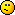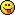# bybb

This is a challenge for yourself to make the shortest FizzBuzz you can!
Here's my one in Python 3:
```[(str(i) if (i%3 and i%5) else "")+("Fizz" if not i%3 else "")+("Buzz" if not i%5 else "") for i in range(1, 100)]
```
I'm interested in the different language people will choose.

# Wettining

bybb wrote:

–snip–
Wouldn't you have to put
```print
```
in the beginning of your code for it to output the test though?
Or is outputting not really necessary, just the calculation?

# bybb

Wettining wrote:

bybb wrote:

–snip–
Wouldn't you have to put
```print
```
in the beginning of your code for it to output the test though?
Or is outputting not really necessary, just the calculation?
Depends on how you run it.
If you run it as a py files, yes you'd need print.
If you run it in IDLE (or in python) it prints the output by default.

# novice27b

BRB, gonna write my solution in PiedPiper… https://scratch.mit.edu/projects/117951730/

It's been a while, I'm not sure if I have any documentation for it even…

In the meantime, here's my python3 solution:

```["Fizz"*(1-x%3)+"Buzz"*(1-x%5)or str(x)for x in range(1,100)]
```

# Wettining

`setMy 'Output' ''for i 100 {  if ((i % 3) == 0) {    setMy 'Output' (join 'Fizz' (join ',' (newline)) (toString (my 'Output')))  } ((i % 5) == 0) {    setMy 'Output' (join 'Buzz' (join ',' (newline)) (toString (my 'Output')))  } else {    setMy 'Output' (join (toString i) (join ',' (newline)) (toString (my 'Output')))  }}`

(To run this you have to run the project in GP, download the project here)

# Sheep_maker

JavaScript:
```new Array(100).fill('').map((v,i)=>(++i%3?v:'Fizz')+(i%5?v:'Buzz')||i)
```

# Wettining

C:
```#include <stdio.h>
int main(boop)
{
int i;
for(i = 1; i <= 100; ++i)
{
if (i % 3 == 0)
printf("Fizz");
if (i % 5 == 0)
printf("Buzz");
if ((i % 3 != 0) && (i % 5 != 0))
printf("%d", i);
printf("\n");
}
return 0;
}
```
C++:
```#include <iostream>
int main() {
for (int i = 1; i <= 100; i++) {
if (i%5==0) std::cout << "Buzz" << std::endl;
else if (i%3==0) std::cout << "Fizz" << std::endl;
else std::cout << i << std::endl;
}
return 0;
}
```

# bybb

Wettining wrote:

C:
```#include <stdio.h>
int main(boop)
{
int i;
for(i = 1; i <= 100; ++i)
{
if (i % 3 == 0)
printf("Fizz");
if (i % 5 == 0)
printf("Buzz");
if ((i % 3 != 0) && (i % 5 != 0))
printf("%d", i);
printf("\n");
}
return 0;
}
```
printf is slow cout is better.
`#include <iostream>using namespace std;int main() {	for (int i=1;i<100;i++) {		if (i%3==0)			cout << "Fizz";		if (i%5==0)			cout << "Buzz";		if (i%3&&i%5)			cout << i;		cout << "\n";	}	return 0;}`

# bybb

Wettining wrote:

C:
```#include <stdio.h>
int main(boop)
{
int i;
for(i = 1; i <= 100; ++i)
{
if (i % 3 == 0)
printf("Fizz");
if (i % 5 == 0)
printf("Buzz");
if ((i % 3 != 0) && (i % 5 != 0))
printf("%d", i);
printf("\n");
}
return 0;
}
```
C++:
```#include <iostream>
int main() {
for (int i = 1; i <= 100; i++) {
if (i%5==0) std::cout << "Buzz" << std::endl;
else if (i%3==0) std::cout << "Fizz" << std::endl;
else std::cout << i << std::endl;
}
return 0;
}
```
That fizzbuzz isn't correct since it will never output “FizzBuzz” on one line and also std::endl causes a delay just use \n in the string# MegaApuTurkUltra

bybb wrote:

also std::endl causes a delay
“a delay”

# bybb

MegaApuTurkUltra wrote:

bybb wrote:

also std::endl causes a delay
“a delay”
https://stackoverflow.com/a/35581029
stream << std::endl is actually stream << ‘\n’ << std::flush. Explicit flushing has performance drawbacks, and this is why you shouldn't use it in performance-critical situations. You rarely think about such negligible performance issues while debugging, so it actualy is a good practice to explicitly flush debug output.

# MegaApuTurkUltra

bybb wrote:

MegaApuTurkUltra wrote:

bybb wrote:

also std::endl causes a delay
“a delay”
snip
Sorry, I assumed you didn't actually know what endl was actually doing when you said it was just “a delay” so I was commenting on that. Looks like you do know what you're talking about though# bybb

MegaApuTurkUltra wrote:

bybb wrote:

MegaApuTurkUltra wrote:

bybb wrote:

also std::endl causes a delay
“a delay”
snip
Sorry, I assumed you didn't actually know what endl was actually doing when you said it was just “a delay” so I was commenting on that. Looks like you do know what you're talking about thoughI first discovered it had some sort of delay when i was making a C++ program I could call from within a batch file to print coloured text within Windows, any text printed without the newline argument passed to my program would complete and print the next bit of text instantly, but when the newline flag was passed it had a delay, I did some research and learnt about how it was the equivalent of a newline and a buffer flush, rather than just a newline.

This is one of the best examples of my why I love programming so much, you can be doing something just for fun and end up learning half a dozen new things you wouldn't have otherwise.

# xn--cr8h

bybb wrote:

```[(str(i) if (i%3 and i%5) else "")+("Fizz" if not i%3 else "")+("Buzz" if not i%5 else "") for i in range(1, 100)]
```
you can remove a fair bit of whitespace from that…

anyway here's mine in Dolphin Smalltalk 7 (179 bytes)
```Transcript show.1to:100do:[:i|Transcript show:(i\\3=0ifTrue:['Fizz'])displayString;show:(i\\5=0ifTrue:['Buzz'])displayString;show:(((i\\3>0)and:[i\\5>0])ifTrue:[i])displayString;cr]
```

ungolfed:
```Transcript show.
1 to: 100 do:
[:i |
Transcript
show: (i \\ 3 = 0 ifTrue: ['Fizz']) displayString;
show: (i \\ 5 = 0 ifTrue: ['Buzz']) displayString;
show: (((i \\ 3 > 0) and: [i \\ 5 > 0]) ifTrue: [i]) displayString;
cr
]
```

if we remove the need to show the transcript and print newlines we can reduce it to 161 bytes!

```1to:100do:[:i|Transcript show:(i\\3=0ifTrue:['Fizz'])displayString;show:(i\\5=0ifTrue:['Buzz'])displayString;show:(((i\\3>0)and:[i\\5>0])ifTrue:[i])displayString]
```

edit: this is shorter

```Transcript show.1to:100do:[:i|Transcript show:(i\\15=0ifTrue:['FizzBuzz']ifFalse:[i\\5=0ifTrue:['Buzz']ifFalse:[i\\3=0ifTrue:['Fizz']ifFalse:[i displayString]]]);cr]
```

# Wettining

bybb wrote:

Wettining wrote:

C:
```#include <stdio.h>
int main(boop)
{
int i;
for(i = 1; i <= 100; ++i)
{
if (i % 3 == 0)
printf("Fizz");
if (i % 5 == 0)
printf("Buzz");
if ((i % 3 != 0) && (i % 5 != 0))
printf("%d", i);
printf("\n");
}
return 0;
}
```
printf is slow cout is better.
`#include <iostream>using namespace std;int main() {	for (int i=1;i<100;i++) {		if (i%3==0)			cout << "Fizz";		if (i%5==0)			cout << "Buzz";		if (i%3&&i%5)			cout << i;		cout << "\n";	}	return 0;}`
It's C not C++ lol

# bybb

I wanted to do it in Batch but I didn't realise Batch actually had a modulo operator… Oh well.
`@echo offgoto start:moduloset /a return=%1set /a divide=%2:loop_2if %return% gtr 0 (set /a return=%return%-%divide%goto loop_2)exit /b:startset /a i=1set /a return=0:loop_1set "fb="set setted=0call :modulo %i% 3if %return% equ 0 (set fb=%fb%Fizzset setted=1)call :modulo %i% 5if %return% equ 0 (set fb=%fb%Buzzset setted=1)if %setted% neq 1 set fb=%i%echo %fb%set /a i=%i%+1if %i% equ 101 goto eol_1goto loop_1:eol_1exit /b`

# MegaApuTurkUltra

bybb wrote:

I wanted to do it in Batch but I didn't realise Batch actually had a modulo operator… Oh well.
Just Windows Things™

```#!/bin/bash
for i in {1..100}; do
case "\$((i % 3))\$((i % 5))" in
00) echo FizzBuzz;;
0*) echo Fizz;;
*0) echo Buzz;;
*) echo \$i
esac
done
```

# TheAspiringHacker

bybb wrote:

Wettining wrote:

C:
```#include <stdio.h>
int main(boop)
{
int i;
for(i = 1; i <= 100; ++i)
{
if (i % 3 == 0)
printf("Fizz");
if (i % 5 == 0)
printf("Buzz");
if ((i % 3 != 0) && (i % 5 != 0))
printf("%d", i);
printf("\n");
}
return 0;
}
```
printf is slow cout is better.
`#include <iostream>using namespace std;int main() {	for (int i=1;i<100;i++) {		if (i%3==0)			cout << "Fizz";		if (i%5==0)			cout << "Buzz";		if (i%3&&i%5)			cout << i;		cout << "\n";	}	return 0;}`
You can make this shorter by replacing `using namespace std` with `using std::cout`.

# xn--cr8h

TheAspiringHacker wrote:

bybb wrote:

Wettining wrote:

C:
```#include <stdio.h>
int main(boop)
{
int i;
for(i = 1; i <= 100; ++i)
{
if (i % 3 == 0)
printf("Fizz");
if (i % 5 == 0)
printf("Buzz");
if ((i % 3 != 0) && (i % 5 != 0))
printf("%d", i);
printf("\n");
}
return 0;
}
```
printf is slow cout is better.
`#include <iostream>using namespace std;int main() {	for (int i=1;i<100;i++) {		if (i%3==0)			cout << "Fizz";		if (i%5==0)			cout << "Buzz";		if (i%3&&i%5)			cout << i;		cout << "\n";	}	return 0;}`
You can make this shorter by replacing `using namespace std` with `using std::cout`.
you can also make this shorter by not using 8 HECKING SPACES to indent

# Sheep_maker

xn--cr8h wrote:

TheAspiringHacker wrote:

snip
you can also make this shorter by not using 8 HECKING SPACES to indent
Those are tab characters, not sets of 8 spaces.